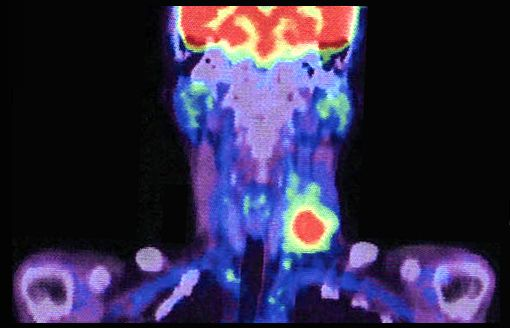# What are the Uses of Nuclear Radiation

Nuclear Radiation has several different applications and, in this article, we will look at several such uses of nuclear radiation. In particular, we will look at radiocarbon dating and the use of radioisotopes in medicine.

Radiocarbon dating  is a method for determining the age of the dead organic material, developed by Willard Libby in the late 1940s. For this, he was awarded the Nobel Prize for Chemistry in 1960. The method makes use of the decay of carbon-14 to determine when the organism making up the material had died.

In the upper atmosphere, cosmic rays interact with various molecules, which lead to the production of many neutrons. These neutrons, in turn, react with atoms of nitrogen gas, producing the unstable isotope carbon-14 in the following reaction:$\mathrm{_{7}^{14}N}+{}_{0}^{1}n\rightarrow\mathrm{_{6}^{14}C}+{}_{1}^{1}H$

Carbon-14 is an unstable isotope of carbon. It undergoes beta minus decay, producing nitrogen-14 again:$\mathrm{_{6}^{14}Au}\rightarrow\mathrm{_{7}^{14}N}+_{-1}^{0}e+_{0}^{0}\bar{\nu_{e}}$

The above process has a half life of 5730 years.

The ratio of carbon-14 to cabon-12 in the atmosphere remain the same. The carbon-14 in the atmosphere end up in molecules of carbon dioxide. Since living things are constantly taking in carbon, the proportion of carbon-14 to carbon-12 in their bodies become the same as the proportion of carbon-14 to carbon-12 in the atmosphere.

When living things die, they stop taking in carbon. The carbon-14 in their bodies now continue to decay, and it is replenished no longer. So, after death, the proportion of carbon-14 to carbon-12 in  the body of a once-living organism continues to diminish.

Since we know the half life of carbon and the ratio of carbon-14 to carbon-12 in a living organism, if we can measure the activity of carbon-14 decay from a dead body we can then calculate how long the organism has been dead for. The technique can be applied to find when anything made from living material was constructed—including materials such as wood and fabric.

Famous cases of radiocarbon dating include “Ötzi the Iceman” (the remains of a dead person buried about 5000 years ago), the “Shroud of Turin“, and the Dead Sea Scrolls.

Radiocarbon dating is not perfect. The composition of carbon dioxide in the atmosphere has changed slightly over the years. In addition, carbon dating may not be accurate when attempting to date things that are more than about 40 000 years old. This is because the proportion of carbon-14 remaining is too low to get an accurate reading of the activity.

Dating with Potassium-40

For finding the age of objects which are much older, the decay of potassium-40 to argon-40 can be used. The beta plus decay process:$\mathrm{_{19}^{40}K}\rightarrow\mathrm{_{18}^{40}Ar}+_{1}^{0}e^{+}+_{0}^{0}\nu_{e}$

has a half life of about 1.25×109 years. Therefore, this is much more suitable than carbon dating for determining the age of much older objects (for instance, to find out when rocks were formed).

Example

The activity of a sample from Ötzi the Iceman was measured to be 0.13 Bq per gram. Given that the activity of living tissue is about 0.23 Bq per gram, find how long ago Ötzi the Iceman was alive.

First we will find the decay constant:$\lambda=\frac{\mathrm{ln\:2}}{t_{1/2}}=\frac{\mathrm{ln\:2}}{5730\times 365\times 24\times\ 60\times\ 60}=3.84\times 10^{-12}\mathrm{\:s^{-1}}$.

Then,$A=A_0e^{-\lambda t}\Rightarrow \frac{A}{A_0}=e^{-\lambda t}$.

Taking ln of both sides, we have,$\mathrm{ln\:}\left( \frac{A}{A_0}\right)=-\lambda t\Rightarrow t=\frac{1}{\lambda}\frac{A}{A_0}$.

Then,$t=\frac{1}{3.84\times 10^{-12}}\times \frac{0.13}{0.23}=1.47\times10^{11}\mathrm{\:s}\approx\mathrm{4700\:years}$.

## Uses of Nuclear Radiation in Medicine

Nuclear radiation is put to many different uses in medicine, for both diagnosis and therapy.

Meta-stable tachnitium-99 is an isotope of Technitium ($_{43}^{99}\mathrm{Tc}$) which is produced during the$\beta^{-}$ decay of Molybdenum-99 ($_{42}^{99}\mathrm{Mb}$). The nucleus of$_{43}^{99}\mathrm{Tc}$ formed is in an excited state, and it decays by emitting a$\gamma$ ray. The$\gamma$ decay of meta-stable technitium-99 has a half life of about 6 hours, which is much longer than half-lives of typical$\gamma$ decays. This is ideal, since it takes some time for the body cells to absorb any material injected. The injected$_{43}^{99}\mathrm{Tc}$ is taken up by cancerous cells (they do not enter healthy cells), where they undergo$\gamma$ decay. Using a gamma camera, the position of cancerous cells could be detected.

Iodine-131 is an unstable isotope that is used to destroy cancer cells in the thyroid gland.

Positron-Electron Tomography (PET) scanning also makes use of nuclear radiation. Here,  molecules containing atoms that undergo$\beta^{+}$ decay is introduced into the body. The$\beta^{+}$particles are positrons ($e^{+}$) and they annihilate when they come into contact with electrons ($e^{-}$). The annihilation produces a pair of$\gamma$ photons, which can  then be detected.One of the Uses of Nuclear Radiation – A PET Scan

###### PET Scan Image originally by Akira Kouchiyama, [CC BY-SA 3.0]